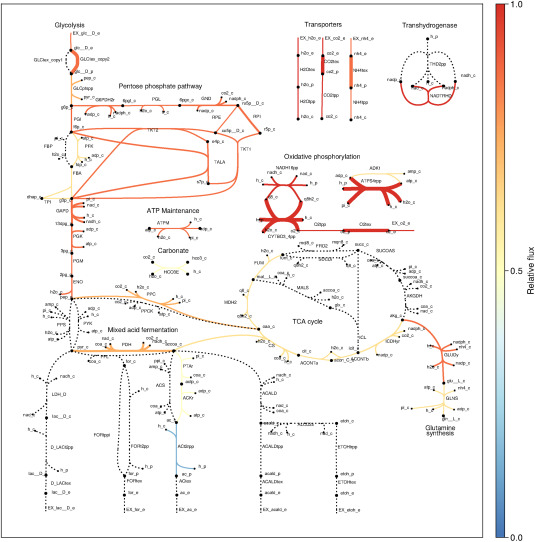Intranet

New publication: interrogating the effect of enzyme kinetics by constraint-based metabolic modeling

PerMedCoE researchers participated in implementing a new method using the previously developed COBREXA.jl which unlocks the ability to calculate the sensitivities of predicted reaction fluxes and enzyme concentrations to turnover numbers in enzyme-constrained metabolic models. This new method shows that optimal solutions of constraint-based metabolic models problems can be efficiently differentiated.

The paper, ‘Interrogating the effect of enzyme kinetics on metabolism using differentiable constraint-based models’, adds important insights into how this method can be generalized to arbitrarily complex models and how differentiating the optimal solution of a model that incorporates thermodynamic and kinetic rate equations explains the effect of metabolite concentrations on biomass growth. It also benchmarks metabolite sensitivities against a large experimental gene knockdown study and identifies several applications of differentiating optimal solutions of constraint-based metabolic models and how they connect to classic metabolic control analysis

### COBREXA.jl & metabolic models

Metabolic models are typically characterised by a large number of parameters. Traditionally, metabolic control analysis is applied to differential equation-based models to investigate the sensitivity of model predictions to parameters. A corresponding method for constraint-based models was lacking due to their formulation as optimization problems. PerMedCoE had developed a Julia language package COBREXA.jl that allows high-performance analysis of the constraint-based metabolic models.Figure shows predicted reaction fluxes and enzyme concentrations of Escherichia coli growing aerobically on glucose in minimal media using the GECKO formulation (Sánchez et al., 2017) of the metabolism, together with turnover numbers for all the metabolic enzymes.

### Key findings

Differentiating the optimal solutions of constraint-based metabolic models has opened the door to more sophisticated, gradient-based analyses. Improved estimates of model parameters that can be generated by the method will be used to enhance the capabilities and precision of simulation methodologies that depend on the exact reproduction of cell metabolism. To sum up:

• Solutions of convex optimization problems can be efficiently differentiated.
• Metabolic control analysis can be applied to constraint-based models.
• Differentiable constraint-based models can be used for parameter estimation.
• Automatic differentiation can be leveraged to find sensitivities of complex models